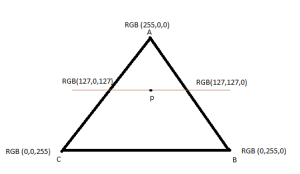suda Senior Java EE Develope specialises in Pdf forms , Fonts, application servers and Image manipulation, meditates in spare time.

# PDF Gouraud shading (Free-Form) Color Interpolation

At IDR Solutions I spend a lot of time working with PDF as I’m involved in the development of our Java PDF Library and PDF to HTML5 Converter.

I thought that this article would be useful to illustrate the algorithm that is used to shade Free-form meshes in PDF files and how to find the color intensity at any given point in a triangular mesh.

Case Example : Find the color intensity of Point P.This task can be accomplished by applying linear interpolation; In order to find this intensity, the cord points and colors of each vertex points needs to be known in advance. Coordinate points are defined in Bites Per Coordinate values and color vertex points  are defined in bits per component values. Please refer to Decode Array to find the number of components that is used to represent the coordination points mapping area and color mapping area; in Decode array these ranges are specified as follows…. [xmin xmax ymin ymax c1,min c1,max … cn,min cn,max]

once the coordinate points and colors of each vertex is known then a horizontal line need to be drawn across point “P”. This line is also referred as “Scan Line”. Coordination points where the scan line intersects in edges AC and AB has to be calculated (this can be accomplished by calculating the distance percentage of each cross section) in order to find the scan intersection point color values.

Once the intersection point colors are known then point “P” color can easily be calculated by calculating the distance of P across the line and mapping it with color deviation.

I Hope you find this information useful.

## Are you a Developer working with PDF files?suda Senior Java EE Develope specialises in Pdf forms , Fonts, application servers and Image manipulation, meditates in spare time.

## How to insert an image into a PDF

Recently, we released JPedal 2023.07 which contains the ability to insert images into PDF files. All you need is a copy of JPedal, a...